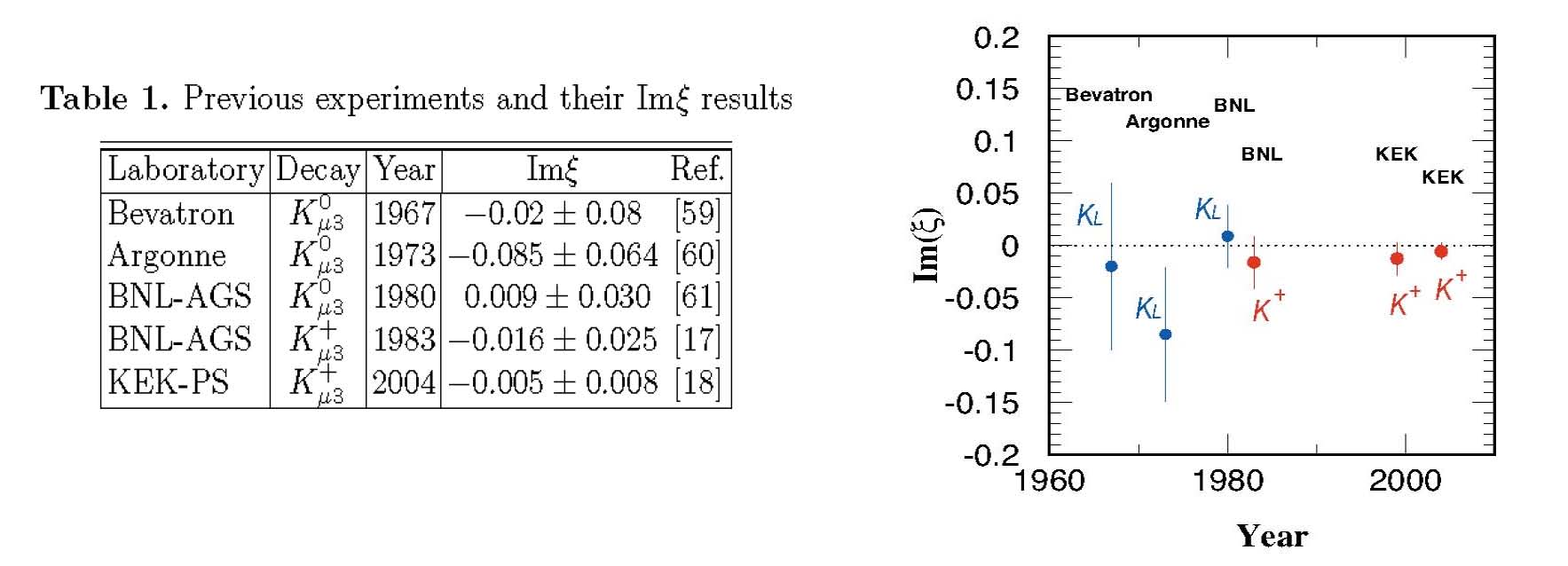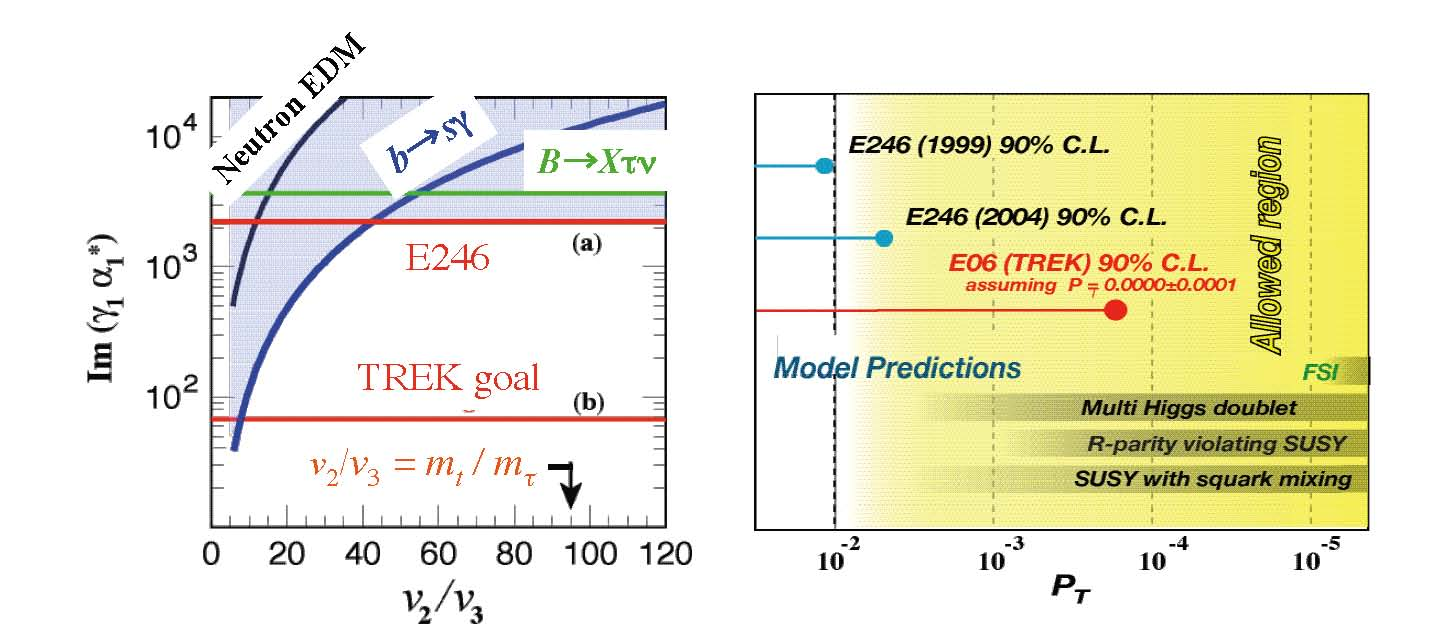# Muon Transverse Polarization (PT)

## PT as a T-odd quantity

In the three-body decay of Kμ3 a decay plane is defined in the center-of-mass system. Muon transverse polarization (PT) is the polarization component perpendicular to this decay plane. Mathematically it can be expressed as a correlation of the three vectors of the muon spin (σμ), the pion momentum (pπ) and the muon momentum (pμ). Since all the involved vectors change sign when time is reversed, this correlation, namely PT, has a T-odd character. Under the condition that spurious effects from final state interactions are negligibly small, a non-vanishing value of PT is a clear evidence for T violation.## Feature of PT

The transverse muon polarization (PT) in K+→ π0 μ+ ν (Kμ3) decays with T-odd correlation was suggested by J.J.Sakurai about 50 years ago to be a clear signature of T violation. Unlike other T-odd channels in e.g. nuclear beta decays, PT in Kμ3 has the advantage that the final state interactions (FSI), which may mimic T violation by inducing a spurious T-odd effect, are very small. This argument applies most particularly to K+μ3 decay with only one charged particle in the final state where the FSI contribution is only from higher order loop levels and is calculable. Thus, it is not surprising that, over the last two decades, dedicated experiments have been carried out in search of non-zero PT in Kμ3 decays. An important feature of a PT study is the fact that the contribution to PT from the Standard Model (SM) is nearly zero (∼ 10-7 ). Therefore, in a PT search we are investigating new physics beyond the SM.## Experimental status of PT

The most recent research of PT has been performed at KEK as the E246 experiment. This experiment was carried out by an international collaboration whose core members continue the current J-PARC TREK experiment. The E246 result was consistent with no T violation but provided the world best limit of
PT = -0.0017 ± 0.0023(stat) ± 0.0017(syst)
and constrained the parameter spaces of several contender models. It was, however, statistics limited, mainly due to insufficient accelerator beam intensity in spite of smaller systematic errors. Now we intend to continue the PT experiment further at J-PARC where higher accelerator beam intensity will be available and a higher experimental sensitivity is promised, in order to search for new physics beyond SM. We aim for a sensitivity of ΔPT ∼ 10-4 [TREK proposal].## PT in the Standard Model

In order to formulate physics motivation for this experiment, we look at what is predicted in the SM for PT. A T-violating (or CP-violating) amplitude arises from the relative phases between diagrams or complex coupling constants in a diagram. Since only a single element of the CKM matrix Vus is involved for the W - exchanging semi-leptonic Kμ3 decay in the SM, no CP violation appears in the first order. This is a general feature for vector (and axial vector) type interactions. The SM contribution comes from only higher order effects. The possible size of its contribution was once suggested qualitatively in to be less than PT < 10-6. An actual value based on the lowest-order vertex radiative corrections to the u γμ (1 - γ5) s Wμ vertex was presented in the textbook of Bigi and Sanda (CP violation). This has been estimated to be less than 10-7. This fact constitutes the main motivation of the physics background for PT experiment as a search for new physics. Since the effect arising from FSI is known to be of the order of 10-5 and it is calculable, an observation of a non-zero PT implies unambiguously the existence of CP violation mechanisms beyond the SM, namely new physics.

## Theoretical models

The theoretical studies of PT in various models of new physics have been of interest for many years and a number of calculations have been done. As candidate theories to give rise to sizable PT, multi-Higgs models were studied. In the 1990s, at the start of the E246 experiment there were renewed calculations in the frame work of the three Higgs doublet model, leptoquark model, or some class of SUSY models such as the R-parity breaking model, which all showed that PT may be as large as 10-3 or even 10-2 without conflicting with other experimental constraints. On the other hand, in the framework of models such as minimal standard or the left-right symmetric model, negligiblly small PT is given. Clearly, an improvement in the measurement of PT will constitute an important tool to constrain these different models. In the three Higgs doublet model the importance of a PT measurement in K+→ μ+νγ as well as in Kμ3 has been pointed out in thory papers.